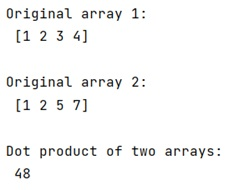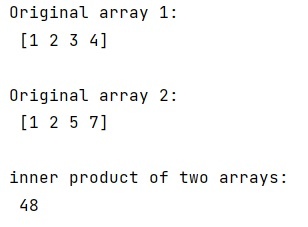# Difference between numpy dot() and inner() Methods

Learn, about the difference between numpy dot() and inner() Methods in Python.
Submitted by Pranit Sharma, on January 25, 2023

NumPy is an abbreviated form of Numerical Python. It is used for different types of scientific operations in python. Numpy is a vast library in python which is used for almost every kind of scientific or mathematical operation. It is itself an array which is a collection of various methods and functions for processing the arrays.

## numpy.dot() Method

The numpy.dot() function is used to find the dot product of two arrays. It returns the dot product of a and b. If a and b are both scalars or both 1-D arrays then a scalar is returned; otherwise, an array is returned. If out is given, then it is returned.

Let us understand with the help of an example,

## Python code to demonstrate the example of numpy.dot() Method

```# Import numpy
import numpy as np

# Creating two numpy arrays
arr1 = np.array([1, 2, 3, 4])
arr2 = np.array([1, 2, 5, 7])

# Display original arrays
print("Original array 1:\n",arr1,"\n")
print("Original array 2:\n",arr2,"\n")

# Finding dot product
res = np.dot(arr1,arr2)

# Display the result
print("Dot product of two arrays:\n",res)
```

Output:## numpy.inner() Method

The numpy.inner() is used to find an inner product of two arrays. It finds the ordinary inner product of vectors for 1-D arrays (without complex conjugation), in higher dimensions a sum product over the last axes.

If a and b are two arrays, it returns a ndarray. If a and b are both scalars or both 1-D arrays then a scalar is returned; otherwise, an array is returned. out.shape = (*a.shape[:-1], *b.shape[:-1]).

Let us understand with the help of an example

## Python code to demonstrate the example of numpy.inner() Method

```# Import numpy
import numpy as np

# Creating two numpy arrays
arr1 = np.array([1, 2, 3, 4])
arr2 = np.array([1, 2, 5, 7])

# Display original arrays
print("Original array 1:\n",arr1,"\n")
print("Original array 2:\n",arr2,"\n")

# Finding inner product
res = np.inner(arr1,arr2)

# Display the result
print("inner product of two arrays:\n",res)
```

Output: